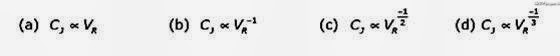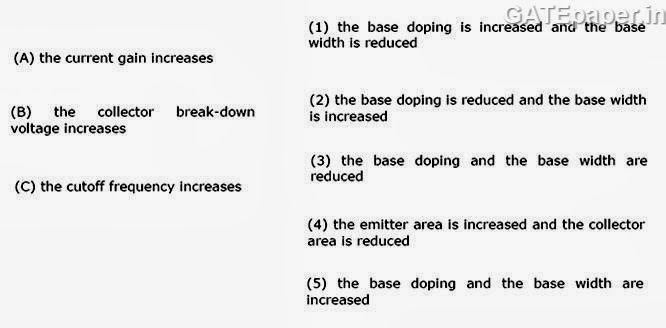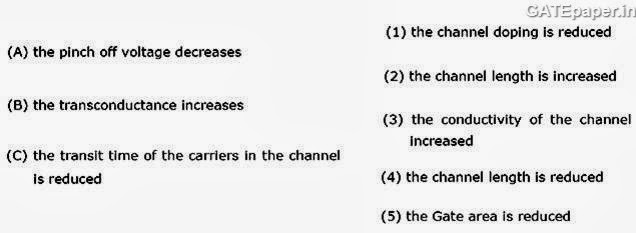### GATE 1995 Video Solution on EDC (Electronic Devices and Circuits)

1. The drift velocity of electrons, in silicon
a. Is proportional to the electric field for all values of electric field
b. Is independent of the electric field
c. Increases at low values of electric field and decreases at high values of electric field exhibiting negative differential resistance.
d. Increases linearly with electric field at low values of electric field and gradually saturates at higher values of electric field.

2. The diffusion potential across a PN junction
a. Decreases with increasing doping concentration
b. Increases with decreasing band gap
c. Does not depend on doping concentration
d. Increases with increase in doping concentrations

3. The breakdown voltage of a transistor with its base open is BVCEO, that with emitter open is BVCBO then
a. BVCEO = BVCBO
b. BVCEO > BVCBO
c. BVCEO < BVCBO
d. BVCEO not related to BVCBO

4. In a p-type silicon sample, the hole concentration is 2.25 X 1015 cm-3. If the intrinsic carrier concentration is 1.5 X 1010 cm-3, the electron concentration is
a. Zero
b. 1010 cm-3
c. 105 cm-3
d. 1.5 X 1010 cm-3

5. A Zener diode works on the principle of
a. Tunneling of charge carriers across the junction
b. Thermionic emission
c. Diffusion of charge carriers across the junction
d. Hopping of charge carriers across the junction

6. A BJT is said to be operating in the saturation region, if
a. Both the junctions are reverse biased
b. Base emitter junction is in reverse biased, and base collector junction is forward biased
c. Base emitter junction is in forward biased, and base collector junction is reverse biased
d. Both the junctions are forward biased

7. The depletion capacitance, CJ, of an abrupt PN junction with constant doping on either side varies with reverse bias, VR as8. The Ebers – Moll model is applicable to
a. Bipolar junction transistors
b. nMOS transistors
c. Unipolar Junction transistors
d. Junction field effect transistors

9. The probability that an electron in a metal occupies the Fermi level at any temperature T is (T > 0oK)
a. 0
b. 1
c. 0.5
d. none

10. A transistor having α = 0.99 and VBE = 0.7 volts, in the circuit shown, then the value of the collector current will be…………11. The circuit shown in figure, supplies power to an 8 Ω loud speaker. The values of IC and VCE for this circuit will beAnswer: 3.4 Amp and 16.4 Volts

12. An n-channel JFET has IDSS = 1 mA and VP = -5 volts. Its maximum Transconductance is ……..

13. In a Bipolar junction transistor, if14. In a JFET, if15. In an extrinsic semiconductor, if4. The area of cross section of the semiconductor is increased
5. The doping concentration is increased

16. Two identical silicon junction diodes, D1 and D2 are connected back to back as shown. The reverse saturation current IS of each diode is 10-8 Amps and the breakdown voltage is 50 volts. Evaluate the voltage VD1 and VD2 across the diode D1 and D2 by assuming KT/q to be 25mV.Answer: -4.98 volts and 0.018 volts

17. Calculate the capacitance of a circular MOS capacitor, of 0.5 mm dia and having a SiO2 layer of 80 mm thickness, under strong accumulation. Assume the relative dielectric constant of SiO2, Ԑr = 4 and Ԑo = 8.854 X 10-14 F/cm. calculate the breakdown voltage of the capacitor if the dielectric strength of SiO2 film is 107 V/cm.

18. The Fermi level of an n-type germanium film is 0.2 eV above the intrinsic Fermi level towards the conduction band. The thickness of the film is 0.5 µm. Calculate the sheet resistance of the film.
Assume :
ni = 1013 cm-3,
µn = 3500 cm2/V-sec,
µp = 1500 cm2/V-sec,
KT/q = 26 mV.